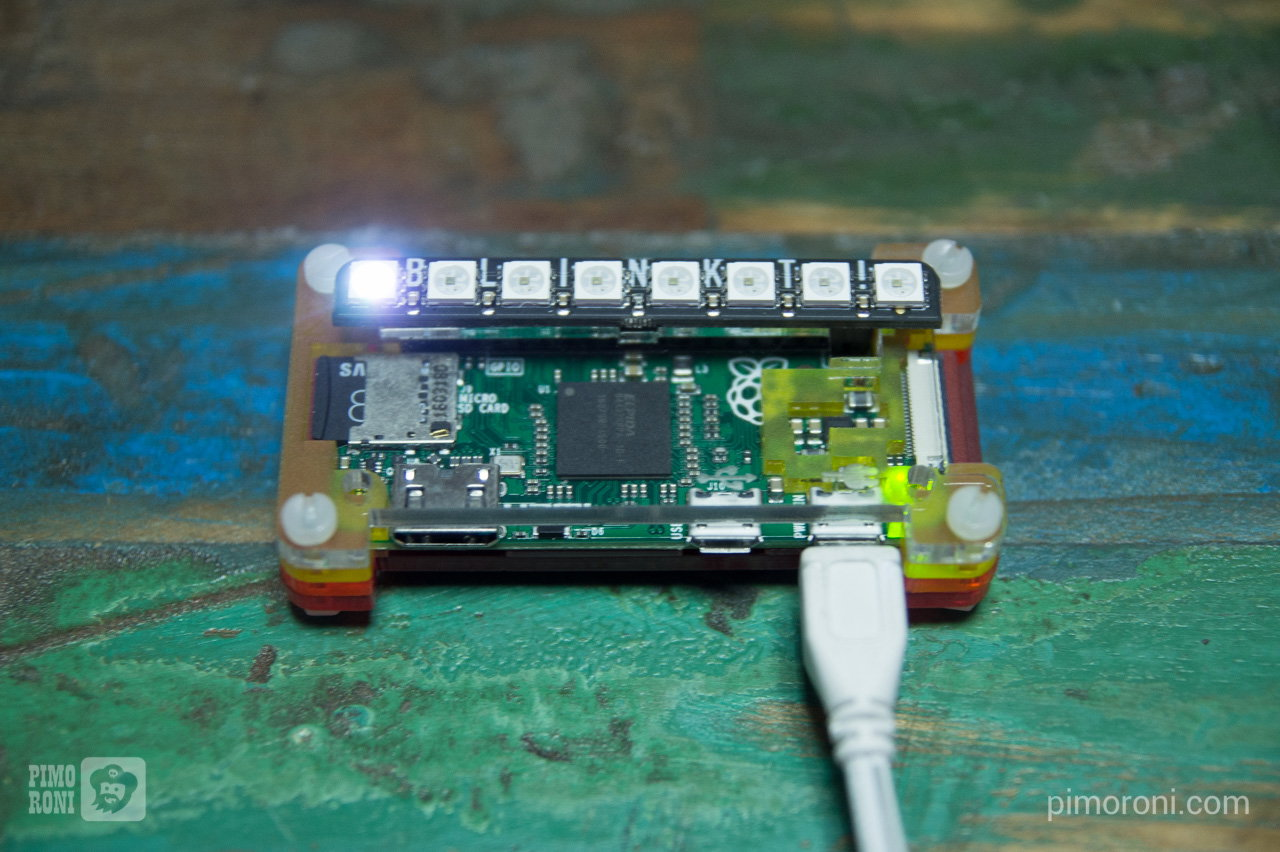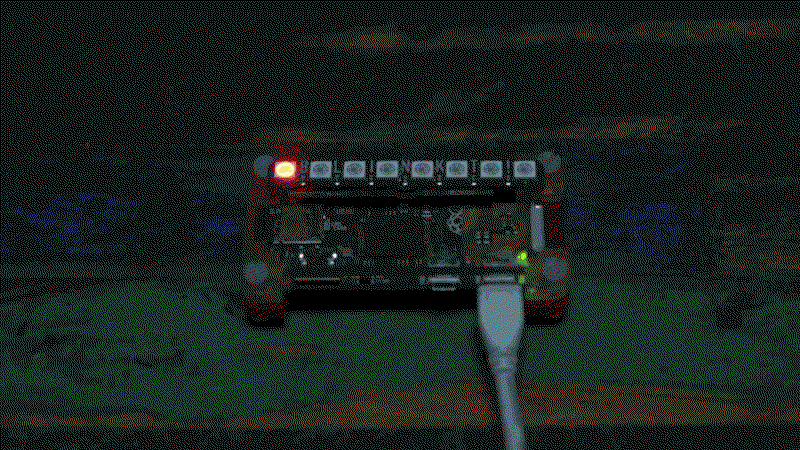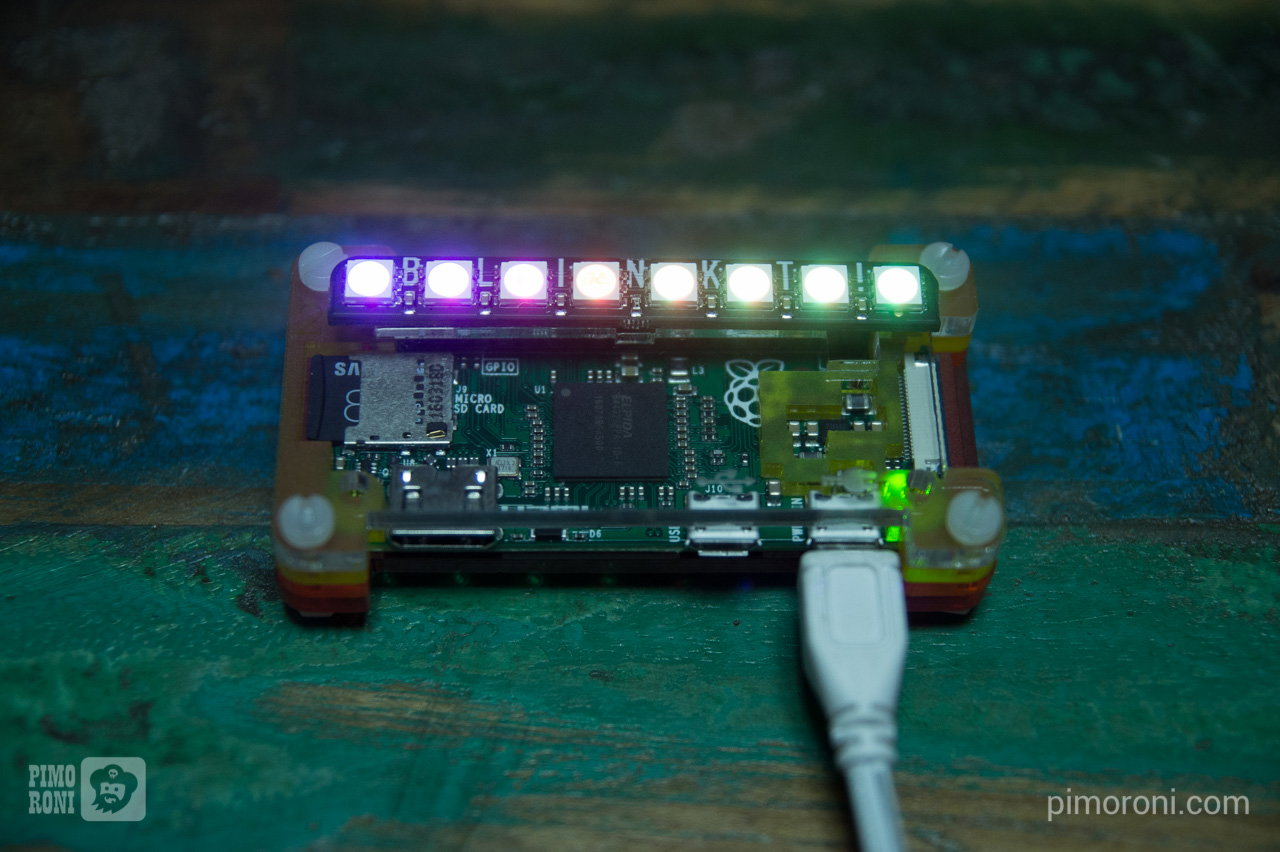This tutorial will show you how to install the Blinkt! Python library, and then walk through its functionality, finishing with an example of how to make a rainbow with Blinkt!

## Installing the software

We always recommend using the most up-to-date version of Raspbian, as this is what we test our boards and software against, and it often helps to start with a completely fresh install of Raspbian, although this isn't necessary.

As with most of our boards, we've created a really quick and easy one-line-installer to get your Blinkt! set up. We'd suggest that you use this method to install the Blinkt! software.

Open a new terminal, and type the following, making sure to type 'y' or 'n' when prompted:

``````curl https://get.pimoroni.com/blinkt | bash
``````

Once that's done, it probably a good idea to reboot your Pi to let the changes propagate.

## Using the software

Open a new terminal and type `python` to open a new Python prompt.

### The set_pixel function

`set_pixel` is the bread and butter function that we use to do everything with Blinkt! Because there are just a single row of eight pixels, it's simple just to iterate through each one in turn, setting its value, and then showing the values on Blinkt! with the `show` function.

`set_pixel` is passed 4 arguments - `x`, `r`, `g` and `b` - with `x` being the pixel position from 0 to 7 on Blinkt! and `r`, `g` and `b` being the RGB colour values.

In the RGB colour system, each colour has a value between 0 and 255, meaning that 255,255,255 is white and 0,0,0 is black. It follows that 255,0,0 is red, 0,255,0 is green, and so on.

Type the following to import the `set_pixel`, `set_brightness`, `show`, and `clear` functions from the `blinkt` library, and then we'll light the pixels different colours. We're also going to set the brightness fairly low, at 0.1, since full brightness is really bright.

``````from blinkt import set_pixel, set_brightness, show, clear

set_brightness(0.1)
``````

First, we'll clear any pixels that might be lit already, then set the leftmost pixel white, and finally call `show()` to display the pixel we set on Blinkt!.

Note that the pixels are indexed from 0 to 7, as is the norm in Python, rather than from 1 to 8, so the leftmost pixel is index 0.

``````clear()
set_pixel(0, 255, 255, 255)
show()
``````Now, we'll do something a little more complex and light the pixels in sequence from left to right, and in red rather than white.

``````import time

while True:
for i in range(8):
clear()
set_pixel(i, 255, 0, 0)
show()
time.sleep(0.05)
``````

Since that's a fair bit of code, we'll break down what it does briefly.

We need to import the `time` library to introduce a small delay between lighting each pixel. The length of this delay sets the animation speed and, in this case, we've picked 0.05 seconds, meaning that our animation will run at 20 frames per second.

We wrap our `for` loop in a `while True` loop, so that it runs constantly. Then, because we have 8 pixels, we use `for i in range(8):` to light each pixel from index 0 to 7 in turn.

In each iteration of the `for` loop, we `clear()` the pixels that were set previously, and then use `set_pixel(i, 255, 0, 0)` followed by `show()` to light the current pixel `i` red. Lastly, we call `show()` to display the set pixel on Blinkt!### Making rainbows

Everyone loves rainbows, right? We're going to make a beautiful rainbow that fades slowly across the eight pixels on Blinkt!

As well as the RGB colour system that we mentioned above, there is the HSV colour system. Whereas RGB explicitly sets both the colour and brightness with just those three red, green and blue values, HSV sets the hue (colour), saturation, value (brightness). This means that you can have things like a dimly lit, light red colour, if you want, by setting HSV to 1.0, 0.2, 0.1, for example.

The hue values in HSV represent colours around a colour wheel. Colour wheels are circular series of colours that begin and end with red at 0 degrees, and then fade through all the colours of the rainbow, through orange, yellow, green, etc.

We're going to use hue values around the colour wheel to make our rainbow, converting the various hue values in degrees to values between 0 and 1, and then converting the HSV colours at full saturation and brightness back into RGB colours that can be passed to Blinkt's `set_pixel` function.

We'll look at all of the code and then go through it bit by bit.

You can type each line of the code below into a Python prompt, but we'd recommend opening a text editor, pasting the code in there, saving it as something like `rainbow.py`, and then running it with `python rainbow.py` in the terminal.

``````import colorsys
import time

from blinkt import set_brightness, set_pixel, show

spacing = 360.0 / 16.0
hue = 0

set_brightness(0.1)

while True:
hue = int(time.time() * 100) % 360
for x in range(8):
offset = x * spacing
h = ((hue + offset) % 360) / 360.0
r, g, b = [int(c * 255) for c in colorsys.hsv_to_rgb(h, 1.0, 1.0)]
set_pixel(x, r, g, b)
show()
time.sleep(0.001)
``````The `colorsys` library is what we'll use to convert our HSV colours to RGB. Again, we'll use `time` to set the animation speed.

We import the functions we'll need from the `blinkt` library, although this time we won't be needing `clear` as we'll be resetting the value of each pixel in every iteration of the loop.

``````from blinkt import set_brightness, set_pixel, show
``````

We're going to define two variables, spacing and hue, that will govern the spacing of the colours of each pixel around the colour wheel. In this case, we've used `360.0 / 16.0`, meaning that our 8 pixels will span half of the colour wheel at any one time. The `hue = 0` means that our rainbow will start at 0 degrees on the colour wheel, although we'll iterate that value through time.

``````spacing = 360.0 / 16.0
hue = 0
``````

`set_brightness(0.1)` sets the overall brightness of Blinkt! to a fairly low value, so that it's not too bright.

Now, we get to out `while True` loop that generates the rainbow.

``````while True:
hue = int(time.time() * 100) % 360
for x in range(8):
offset = x * spacing
h = ((hue + offset) % 360) / 360.0
r, g, b = [int(c * 255) for c in colorsys.hsv_to_rgb(h, 1.0, 1.0)]
set_pixel(x, r, g, b)
show()
time.sleep(0.001)
``````

The hue is calculated by using the time seconds, `time.time()` multiplying it by 100 and then taking the modulus 360, to get a position in degrees around the colour wheel.

Then, we have a loop, `for x in range(8):`, that will let us set each pixel on Blinkt! We use the `spacing` variable that we defined earlier to calculate a hue offset for each pixel, `offset = x * spacing`, and then calculate the individual hue, `h`, for each pixel, adding on the `offset`, taking the modulus 360 again, and then dividing by 360 to get a value between 0 and 1, `h = ((hue + offset) % 360) / 360.0`.

We have to convert our HSV colour to RGB values that can be passed to the `set_pixel` function. We do this with `r, g, b = [int(c * 255) for c in colorsys.hsv_to_rgb(h, 1.0, 1.0)]`, also multiplying each value by 255 and converting to an integer, since the numbers returned by the `colorsys.hsv_to_rgb` function are values between 0 and 1.

``````r, g, b = [int(c * 255) for c in colorsys.hsv_to_rgb(h, 1.0, 1.0)]
``````

The last line of our `for` loop sets the pixel colour,`set_pixel(x, r, g, b)`, and then outside our `for` loop, but still within our `while` loop, we call `show()` and include a small delay, `time.sleep(0.001)`, to set the animation speed. Using a delay of 1 millisecond (0.001 seconds) makes the animation super-smooth.